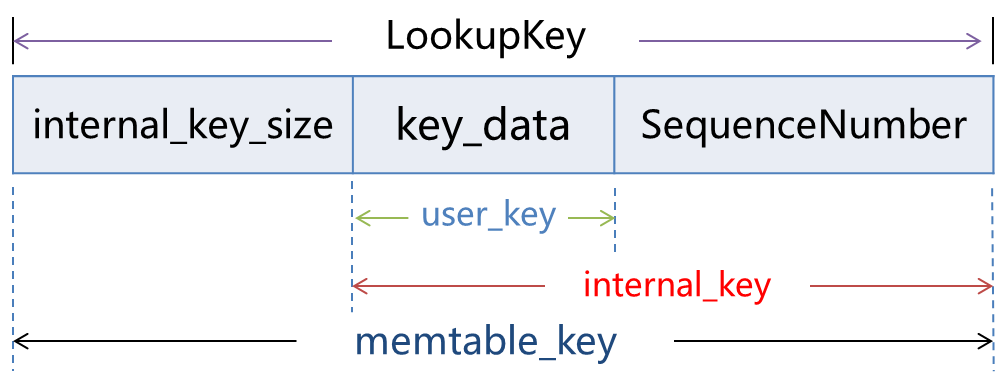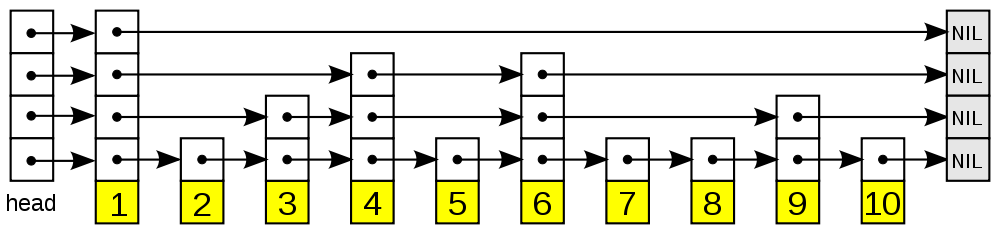# leveldb 笔记六：Memtable 实现

Posted by YuanBao on July 20, 2017

MemTable 结构定义在文件 memtable.h 中，其成员变量如下：

class MemTable {
private:
typedef SkipList<const char*, KeyComparator> Table;

Table table_;
Arena arena_;
KeyComparator comparator_;
int refs_;
...
}


leveldb 中的 MemTable 基于跳表 SkipList 来实现对于键值对的有序管理，通过内存管理器 Arena 来为插入的 key/value 对申请内存，并通过记录引用数量 refs_ 来决定内存释放。

## Memtable 中的 key

1. UserKey：用户提供的键值
2. InternalKey：UserKey + SequenceNumber
3. LookupKey：EncodeString(InternalKey.size()) + InternalKeyenum ValueType {
kTypeDeletion = 0x0,
kTypeValue = 0x1
};


Memtable 的查询接口 Get 传入的是结构 LookupKey，或者称为 Memtable key。这个结构首先将 InternalKey 的长度通过变长字符串进行编码，然后再拼接 InternalKey 形成。可以理解成为带有长度编码的 InternalKey。

memtable 定义了这几种 key 相互转化以及打包的接口，定义在文件 db_format.cc 中。当了解了格式，再去理解实现应该比较容易。

## 跳表 SkipList

Skip lists are a probabilistic data structure that seem likely to supplant balanced trees as the implementation method of choice for many applications. Skip list algorithms have the same asymptotic expected time bounds as balanced trees and are simpler, faster and use less space.leveldb 中的跳表实现非常简洁。跳表的节点 Node 被定义为柔性数组：

template<typename Key, class Comparator>
struct SkipList<Key,Comparator>::Node {
private:
port::AtomicPointer next_;  // flexible array
}


//判断 Key 是否大于节点 Node 中存储的 Key 值
bool KeyIsAfterNode(const Key& key, Node* n) const;

// 寻找第一个大于等于 Key 值的节点，同时将该节点的前置节点存放在数组 prev 中
Node* FindGreaterOrEqual(const Key& key, Node** prev) const;

// 寻找第一个键值小于 key 的节点
Node* FindLessThan(const Key& key) const;


template<typename Key, class Comparator>
typename SkipList<Key,Comparator>::Node*
SkipList<Key,Comparator>::FindGreaterOrEqual(const Key& key, Node** prev)
const {
int level = GetMaxHeight() - 1;  //获得跳表的最高层
while (true) {
Node* next = x->Next(level);   // 从最高层还是查找
if (KeyIsAfterNode(key, next)) {  // 如果当前的节点键值较小，向后移动
x = next;
} else {
if (prev != NULL) prev[level] = x;
if (level == 0) {
return next;
} else {
level--;  // 下降一层继续查找
}
}
}
}


1. 从跳表的最高层链表开始查找，如果当前的节点小于键值 key，那么指针向后移动；
2. 如果当前节点大于等于键值 key，首先记录该节点的前置节点，然后判断当前所在的层数；
3. 如果当前已经抵达最底层，那么直接返回找到的节点，否则降低一层继续查找。

template<typename Key, class Comparator>
void SkipList<Key,Comparator>::Insert(const Key& key) {
Node* prev[kMaxHeight];

// 寻找到插入位置，prev 数组会被填充为该节点在不同层次上的前置节点
Node* x = FindGreaterOrEqual(key, prev);

// 不允许出现相同的键值
assert(x == NULL || !Equal(key, x->key));

int height = RandomHeight();  // 随机计算该节点的高度
if (height > GetMaxHeight()) {
for (int i = GetMaxHeight(); i < height; i++) {
}
max_height_.NoBarrier_Store(reinterpret_cast<void*>(height));
}

x = NewNode(key, height);
for (int i = 0; i < height; i++) {  // 将该节点串接到所有的链表中
x->NoBarrier_SetNext(i, prev[i]->NoBarrier_Next(i));
prev[i]->SetNext(i, x);
}
}


## Memtable 的实现

Memtable 提供了如下几个接口：

void Add(SequenceNumber seq, ValueType type, const Slice& key,
const Slice& value);
bool Get(const LookupKey& key, std::string* value, Status* s);
size_t ApproximateMemoryUsage();
Iterator* NewIterator();
void Ref();
void Unref();


Memtable 本身基于引用计数来管理，当外部使用到该 Memtable 时，其引用计数加一；当没有外部使用者时，该 Memtable 占用的内存将全部被释放。因此对于 Memtable 而言，没有单独释放某个 key/value 内存空间的概念。这也就是为什么 Arena 除了析构函数以外不提供任何内存释放操作的原因。

void MemTable::Add(SequenceNumber s, ValueType type,
const Slice& key,
const Slice& value) {
size_t key_size = key.size();  // user_key
size_t val_size = value.size();
size_t internal_key_size = key_size + 8;  // 计算 InternalKey长度
const size_t encoded_len =
VarintLength(internal_key_size) + internal_key_size +
VarintLength(val_size) + val_size;
char* buf = arena_.Allocate(encoded_len);  // 申请内存
char* p = EncodeVarint32(buf, internal_key_size); // 将InternalKey长度编码
memcpy(p, key.data(), key_size);
p += key_size;
EncodeFixed64(p, (s << 8) | type);  // 填充SequenceNumber 和 Type
p += 8;
p = EncodeVarint32(p, val_size);  // 将 value 长度进行编码
memcpy(p, value.data(), val_size);  // 保存 value 值
assert((p + val_size) - buf == encoded_len);
table_.Insert(buf);  // 插入到跳表中
}


Slice memkey = key.memtable_key();
Table::Iterator iter(&table_);
iter.Seek(memkey.data());


const char* entry = iter.key();  // 当前条目对应的 LookupKey
uint32_t key_length;
// 解析 InternalKey 的长度到 key_length
const char* key_ptr = GetVarint32Ptr(entry, entry+5, &key_length);
// 比较当前条目对应的 user_key 与 参数 key 是否相等
if (comparator_.comparator.user_comparator()->Compare(
Slice(key_ptr, key_length - 8),
key.user_key()) == 0) {
...
}


    const uint64_t tag = DecodeFixed64(key_ptr + key_length - 8);
switch (static_cast<ValueType>(tag & 0xff)) {   // 最后8-bit是valueType
case kTypeValue: {
Slice v = GetLengthPrefixedSlice(key_ptr + key_length);
value->assign(v.data(), v.size());
return true;
}
case kTypeDeletion:
*s = Status::NotFound(Slice());
return true;
}